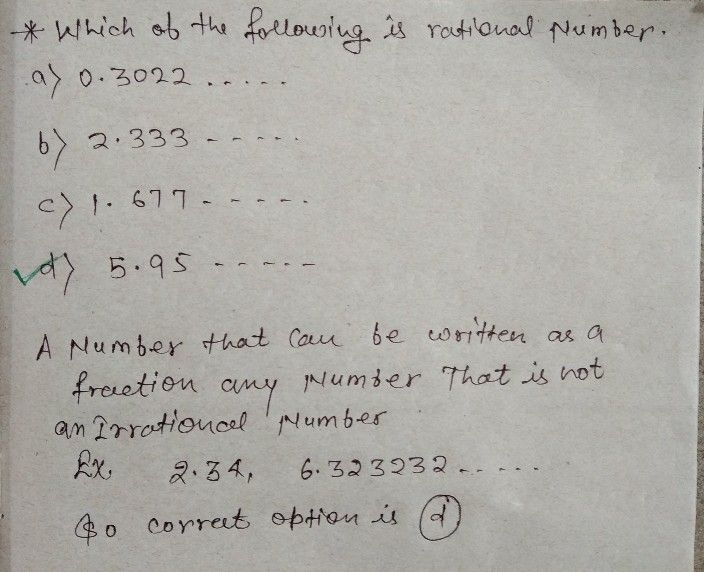Symbol
Problem$1.M.c.0$ $\left(20$ Marks) $\right)$ $14$ 1.Which of the following is rational number. a) $0.3022....$ $b\right)2.333....$ $c\right)1.677....$ d)5.95.. $⌒$ $G$